# Unit Circle: How to Memorize & Use

## What is the Unit Circle?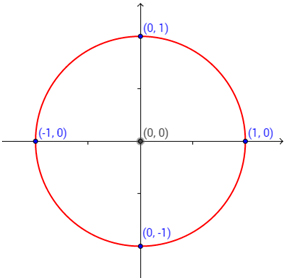The unit circle is a circle, centered at the origin, with a radius of 1.

This circle helps us find the exact values of some trigonometric functions and not the decimal approximations the calculator will give us. For example, it’ll help us find the exact value of sin $latex \frac{\pi}{3}$ or cos30°.

You don’t have to memorize all the values of the trigonometric functions, you just have to understand the unit circle.

## Trigonometry of the Right Triangle and the Unit Circle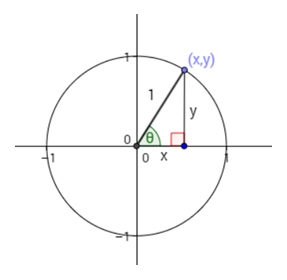Let (x,y) be the point on the circle that is in the first quadrant.

The lengths x and y become the legs of a right triangle whose hypotenuse is actually the radius of our unit circle, i.e. the hypotenuse is 1.

Now let’s use some right angle trigonometry. Note that angle ? is acute.

This shows us that in a unit circle, cos ? = x and sin ? = y, which creates:

Sine is represented by the vertical leg.

Cosine is represented by the horizontal leg.

This formula applies to all the quadrants (it’s not limited to acute angles):

• cos ? is the x coordinate of a point where terminal side of the angle intersects the unit circle
• sin ? is the y coordinate of a point where terminal side of the angle intersects the unit circle
• tan ? =

Using Pythagorean theorem, we have:

But since we have that x = cos ? and y = sin ?, this identity becomes:

This is called the Pythagorean trigonometric identity and it is very useful.

## Trigonometric Functions

If you are asked to find sin60°, you would just need to look up for the y coordinate of the intersecting point on the circle. To find the cosine of the same angle, you just look up for the x coordinate of the same point.

There are some important angles whose sine, cosine and tangent you should memorize. They are given in the following table: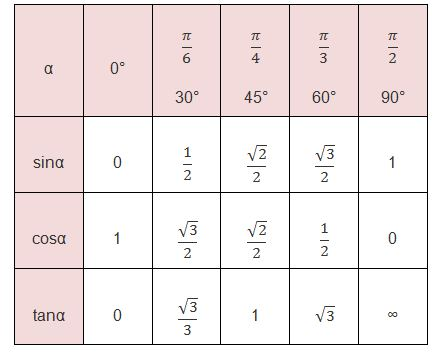This might seem a lot at first, but there’s a trick to help you learn this faster.

## Remembering the Unit Circle

For sine of 30°, 45°, and 60°, you should try to think “1, 2, 3” for the square root number in the numerator. The denominator is 2 and will stay the same.

• sin 30° = or
• sin 45° =
• sin 60° =

For cosine of 30°, 45°, and 60°, you should try to think “3, 2, 1” for the square root number in the numerator. The denominator is 2 and will stay the same.

• cos 30° =
• cos 45° =
• cos 60° = or

So actually, it’s just these three numbers: that you need to memorize.

Tangent will be equal to .

Another way to help you remember the 30° and 60° is the special triangle.

Sketch an equilateral triangle with side length 2. (All sides are 2 and all angles are 60°.)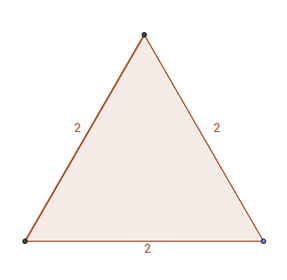Cut it in half. According to Pythagorean theorem, the new side is ?3.Now you can find the sine, cosine and tangent using soh cah toa.  If you have trouble with this concept, please check out our in-depth SohCahToa guide.

SOH = Sin Opposite over Hypotenuse
CAH = Cos Adjacent over Hypotenuse
TOA = Tan Opposite over Adjacent

• sin 30°= (opposite/hypotenuse)=

You can find the values for 60° angle the same way.

The mnemonic ASTC (All Students Take Calculus) can help you remember which ones (sine, cosine, tangent) are positive in which quadrant.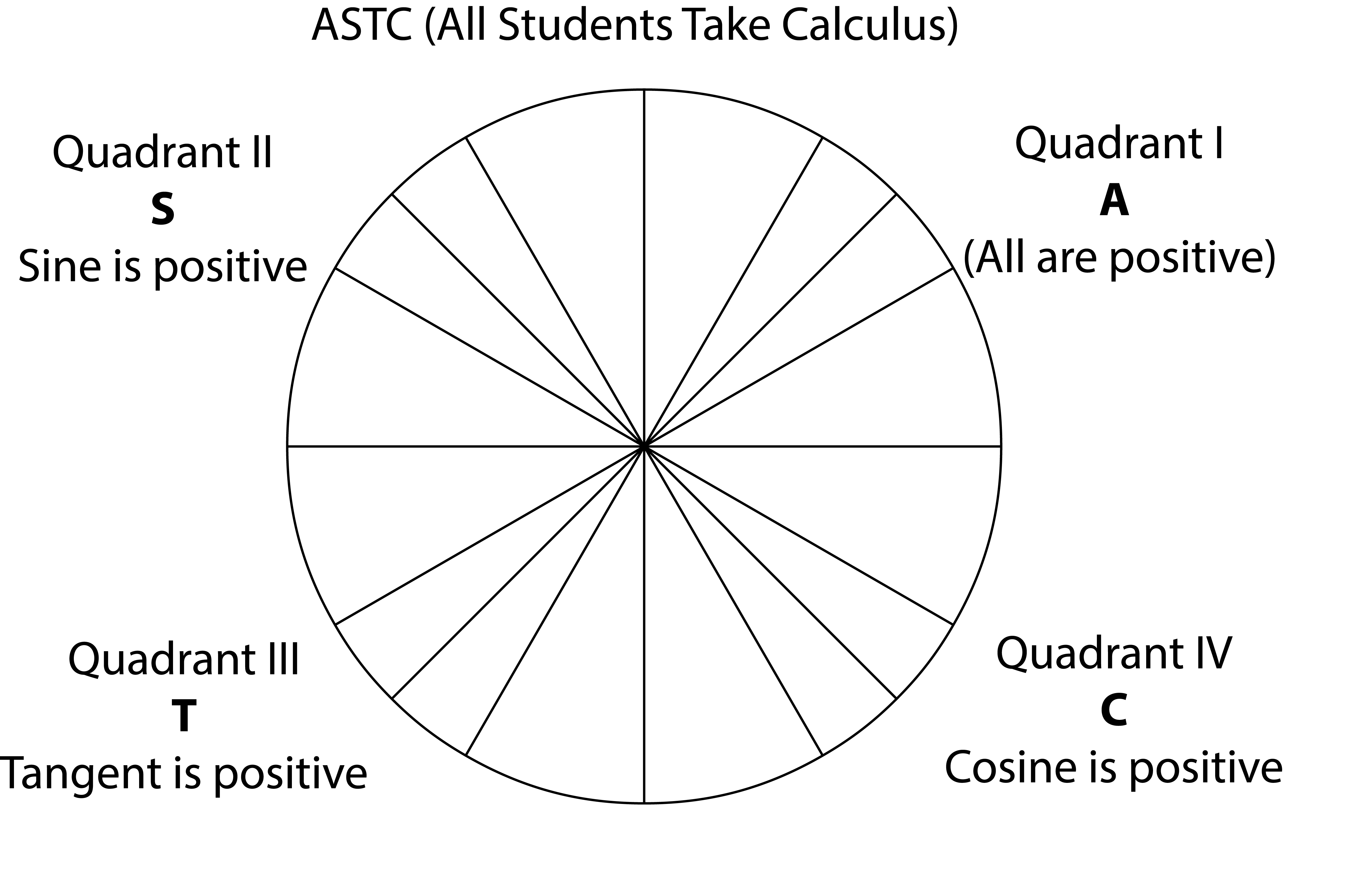• In the first quadrant, All are positive
• In the second quadrant, only Sine is positive
• In the third quadrant, only Tangent is positive
• In the fourth quadrant, only Cosine is positive

[box type=”success” align=”aligncenter” class=”” width=””]Did this help? Learn more topics in Trigonometry and 100+ other subjects at Educator.com, the best place to get in-depth and instant homework help online.[/box]

## Converting Between Radians and Degrees

If you want to convert degrees into radians, simply multiply the number of degrees by .

Solution:

If you want to convert radians to degrees, simply multiply the number of radians by 180/?.

Solution:

=5*60

=300º

## Reference Angle

A reference angle is always positive and is ? 90º. It helps us determine the value of sine, cosine and tangent of an angle that is larger than 90°.

How to find the reference angle?

First sketch the angle ? to see what quadrant it is in. Depending on the quadrant, use these rules to figure out the reference angle:

• Quadrant I: Same as ?

• When the denominator is 6, the reference angle is 30º. Example: 11?/6 reference angle is 30º
• When the denominator is 4, the reference angle is 45º. Example: 5?/4 reference angle is 45º
• When the denominator is 3, the reference angle is 60º. Example: 2?/3 reference angle is 60º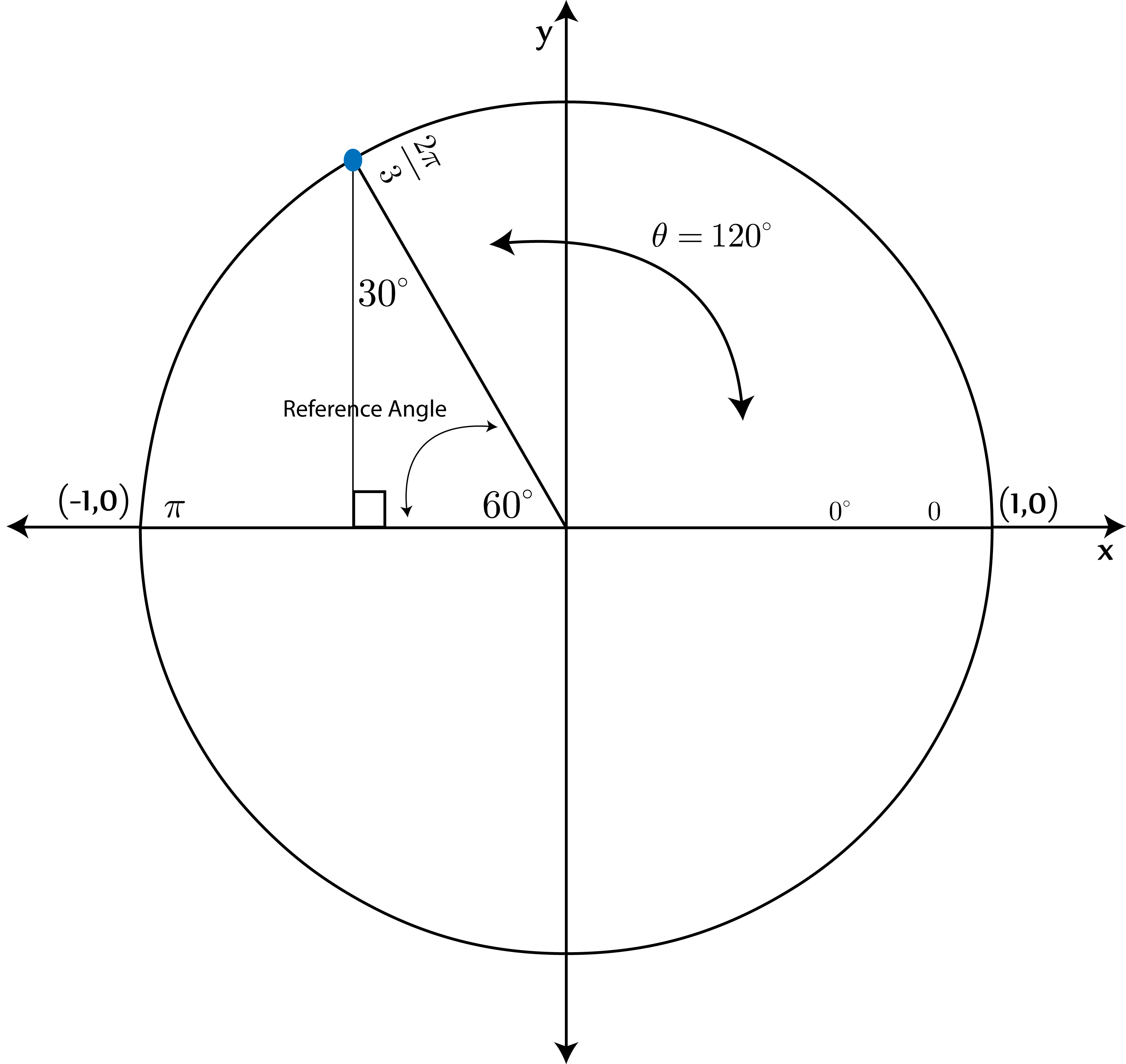## Coterminal Angles

The coterminal angles are angles which, drawn in standard position, share a terminal side. For example angle 30° and angle -330°.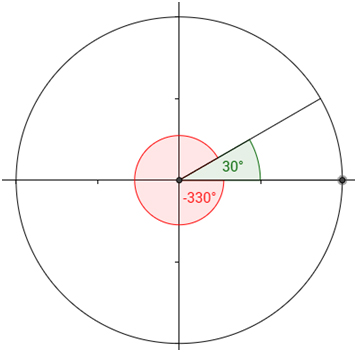The coterminal angles can be both positive and negative. You can sketch the angles and often tell just from looking at them if they are coterminal. Otherwise, for each angle do the following:

• If the angle is positive, keep subtracting 360 from it until the result is between 0 and +360. (In radians, 360° = 2? radians)
• If the angle is negative, keep adding 360 until the result is between 0 and +360.

If the result is the same for both angles, they are coterminal.

These angles are important for us because they have the same value for the trigonometric functions.

Example: Let’s prove that angles 60°, -300° and 420° are all coterminal.

The first angle is 60°

The second one is negative, so let’s add 360: -300°+360°=60°

The last one is positive, so let’s subtract 360: 420°-360°=60°

The result is the same for all angles so, they are coterminal.

## The Unit Circle Chart

Here is a simple unit circle chart that summarizes all the important angles on the unit circle and their sine and cosine values.

Here is a practice unit circle chart that you can use to test your memory.

## Example: Unit Circle for ? in Quadrant I

Let’s do an example problem. What if you have ?=30º and you want to find the cosine and sine for it.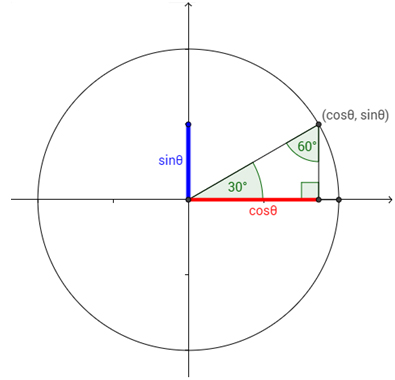We can get the answer straight from the table, or we can use any of the tricks we have mentioned above. Let’s use the first one.

Remember that for sine of 30°, 45° and 60° we use “1, 2, 3” for the square root in the denominator, while the numerator stays 2. For cosine we use “3, 2, 1” and the rest is the same. Our angle is 30°

so we’ll take the first numbers:

## Example: Unit Circle for ? in Quadrant II

What if you have ?=120º and you want to convert it to radians, identify the quadrant, and find its cosine and sine?

Step 1: To convert to radians, simply multiply with ?/180

Step 2: This angle is in the second quadrant (greater than 90° and less than 180°). Using mnemostic ASTC (All Students Take Calculus), we can identify that only sine is positive in this quadrant. Cosine is negative here.

Step 3: When the angle is in Quadrant II, we will subtract it from 180 to figure the reference angle.

180°-120°=60°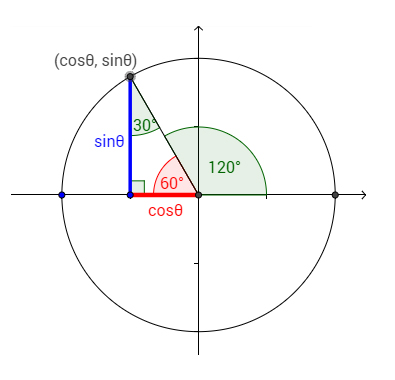Now we can solve for cosine and sine:

## Need More Help?

[box type=”success” align=”aligncenter” class=”” width=””]Learn more topics in Trigonometry and 100+ other subjects at Educator.com, the best place to get in-depth and instant homework help online.[/box]

SPECIAL OFFER!

SUCCESS8